# Empty Set Rewrite Rules

Rules that are marked with a * in the first column are implemented in the latest version of Rodin. Rules without a * are planned to be implemented in future versions. Other conventions used in these tables are described in The_Proving_Perspective_(Rodin_User_Manual)#Rewrite_Rules.

All rewrite rules that match the pattern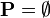$\textbf{P}=\emptyset$ are also applicable to predicates of the form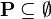$\textbf{P}\subseteq\emptyset$ and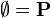$\emptyset=\textbf{P}$, as these predicates are equivalent. All rewrite rules that match the pattern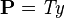$\textbf{P}=\mathit{Ty}$ are also applicable to predicates of the form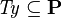$\mathit{Ty}\subseteq\textbf{P}$ and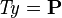$\mathit{Ty}=\textbf{P}$, as these predicates are equivalent.

Name Rule Side Condition A/M
*
DEF_SPECIAL_NOT_EQUAL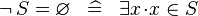$\lnot\, S = \emptyset \;\;\defi\;\; \exists x \qdot x \in S$ where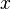$x$ is not free in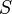$S$ M
*
SIMP_SETENUM_EQUAL_EMPTY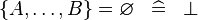$\{ A, \ldots , B\} = \emptyset \;\;\defi\;\; \bfalse$ A
*
SIMP_SPECIAL_EQUAL_COMPSET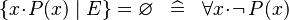$\{ x \qdot P(x) \mid E \} = \emptyset \;\;\defi\;\; \forall x\qdot \lnot\, P(x)$ A
*
SIMP_BINTER_EQUAL_TYPE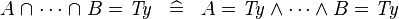$A \binter \cdots \binter B = \mathit{Ty} \;\;\defi\;\; A = \mathit{Ty} \land \cdots \land B = \mathit{Ty}$ where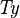$\mathit{Ty}$ is a type expression A
*
SIMP_BINTER_SING_EQUAL_EMPTY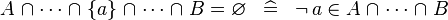$A\binter\cdots\binter\{a\}\binter\cdots\binter B = \emptyset \;\;\defi\;\; \lnot\, a \in A\binter\cdots\binter B$ A
*
SIMP_BINTER_SETMINUS_EQUAL_EMPTY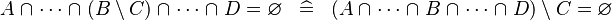$A\binter\cdots\binter(B\setminus C)\binter\cdots\binter D = \emptyset \;\;\defi\;\; (A\binter\cdots\binter B\binter\cdots\binter D) \setminus C = \emptyset$ A
*
SIMP_BUNION_EQUAL_EMPTY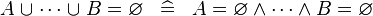$A \bunion \cdots \bunion B = \emptyset \;\;\defi\;\; A = \emptyset \land \cdots \land B = \emptyset$ A
*
SIMP_SETMINUS_EQUAL_EMPTY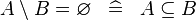$A \setminus B = \emptyset \;\;\defi\;\; A \subseteq B$ A
*
SIMP_SETMINUS_EQUAL_TYPE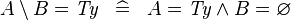$A \setminus B = \mathit{Ty} \;\;\defi\;\; A = \mathit{Ty} \land B = \emptyset$ where$\mathit{Ty}$ is a type expression A
*
SIMP_POW_EQUAL_EMPTY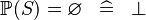$\pow (S) = \emptyset \;\;\defi\;\; \bfalse$ A
*
SIMP_POW1_EQUAL_EMPTY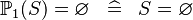$\pown (S) = \emptyset \;\;\defi\;\; S = \emptyset$ A
*
SIMP_KINTER_EQUAL_TYPE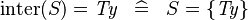$\inter (S) = \mathit{Ty} \;\;\defi\;\; S = \{ \mathit{Ty} \}$ where$\mathit{Ty}$ is a type expression A
*
SIMP_KUNION_EQUAL_EMPTY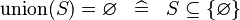$\union (S) = \emptyset \;\;\defi\;\; S \subseteq \{ \emptyset \}$ A
*
SIMP_QINTER_EQUAL_TYPE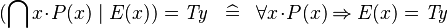$(\Inter x\qdot P(x) \mid E(x)) = \mathit{Ty} \;\;\defi\;\; \forall x\qdot P(x) \limp E(x) = \mathit{Ty}$ where$\mathit{Ty}$ is a type expression A
*
SIMP_QUNION_EQUAL_EMPTY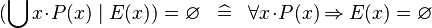$(\Union x\qdot P(x) \mid E(x)) = \emptyset \;\;\defi\;\; \forall x\qdot P(x) \limp E(x) = \emptyset$ A
*
SIMP_NATURAL_EQUAL_EMPTY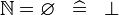$\nat = \emptyset \;\;\defi\;\; \bfalse$ A
*
SIMP_NATURAL1_EQUAL_EMPTY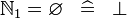$\natn = \emptyset \;\;\defi\;\; \bfalse$ A
*
SIMP_TYPE_EQUAL_EMPTY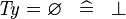$\mathit{Ty} = \emptyset \;\;\defi\;\; \bfalse$ where$\mathit{Ty}$ is a type expression A
*
SIMP_CPROD_EQUAL_EMPTY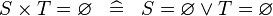$S \cprod T = \emptyset \;\;\defi\;\; S = \emptyset \lor T = \emptyset$ A
*
SIMP_CPROD_EQUAL_TYPE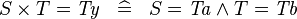$S \cprod T = \mathit{Ty} \;\;\defi\;\; S = \mathit{Ta} \land T = \mathit{Tb}$ where$\mathit{Ty}$ is a type expression equal to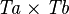$\mathit{Ta} \cprod \mathit{Tb}$ A
*
SIMP_UPTO_EQUAL_EMPTY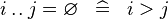$i \upto j = \emptyset \;\;\defi\;\; i > j$ A
*
SIMP_UPTO_EQUAL_INTEGER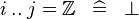$i \upto j = \intg \;\;\defi\;\; \bfalse$ A
*
SIMP_UPTO_EQUAL_NATURAL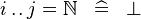$i \upto j = \nat \;\;\defi\;\; \bfalse$ A
*
SIMP_UPTO_EQUAL_NATURAL1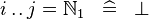$i \upto j = \natn \;\;\defi\;\; \bfalse$ A
*
SIMP_SPECIAL_EQUAL_REL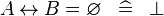$A \rel B = \emptyset \;\;\defi\;\; \bfalse$ idem for operators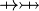$\pfun \pinj$ A
SIMP_TYPE_EQUAL_REL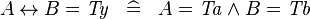$A \rel B = \mathit{Ty} \;\;\defi\;\; A = \mathit{Ta} \land B = \mathit{Tb}$ where$\mathit{Ty}$ is a type expression equal to$\mathit{Ta} \cprod \mathit{Tb}$ A
*
SIMP_SPECIAL_EQUAL_RELDOM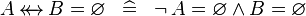$A \trel B = \emptyset \;\;\defi\;\; \lnot\, A = \emptyset \land B = \emptyset$ idem for operator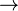$\tfun$ A
SIMP_TYPE_EQUAL_RELDOMRAN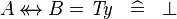$A \trel B = \mathit{Ty} \;\;\defi\;\; \bfalse$ where$\mathit{Ty}$ is a type expression, idem for operator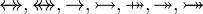$\srel, \strel, \tfun, \tinj, \psur, \tsur, \tbij$ A
*
SIMP_SREL_EQUAL_EMPTY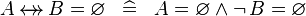$A \srel B = \emptyset \;\;\defi\;\; A = \emptyset \land \lnot\,B = \emptyset$ A
*
SIMP_STREL_EQUAL_EMPTY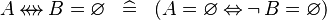$A \strel B = \emptyset \;\;\defi\;\; (A = \emptyset \leqv \lnot\,B = \emptyset)$ A
*
SIMP_DOM_EQUAL_EMPTY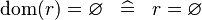$\dom (r) = \emptyset \;\;\defi\;\; r = \emptyset$ A
*
SIMP_RAN_EQUAL_EMPTY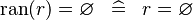$\ran (r) = \emptyset \;\;\defi\;\; r = \emptyset$ A
*
SIMP_FCOMP_EQUAL_EMPTY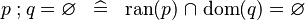$p \fcomp q = \emptyset \;\;\defi\;\; \ran (p) \binter \dom (q) = \emptyset$ A
*
SIMP_BCOMP_EQUAL_EMPTY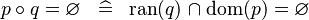$p \bcomp q = \emptyset \;\;\defi\;\; \ran (q) \binter \dom (p) = \emptyset$ A
*
SIMP_DOMRES_EQUAL_EMPTY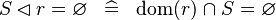$S \domres r = \emptyset \;\;\defi\;\; \dom (r) \binter S = \emptyset$ A
*
SIMP_DOMRES_EQUAL_TYPE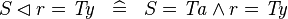$S \domres r = \mathit{Ty} \;\;\defi\;\; S = \mathit{Ta} \land r = \mathit{Ty}$ where$\mathit{Ty}$ is a type expression equal to$\mathit{Ta} \cprod \mathit{Tb}$ A
*
SIMP_DOMSUB_EQUAL_EMPTY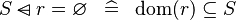$S \domsub r = \emptyset \;\;\defi\;\; \dom (r) \subseteq S$ A
*
SIMP_DOMSUB_EQUAL_TYPE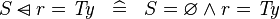$S \domsub r = \mathit{Ty} \;\;\defi\;\; S = \emptyset \land r = \mathit{Ty}$ where$\mathit{Ty}$ is a type expression A
*
SIMP_RANRES_EQUAL_EMPTY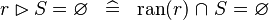$r \ranres S = \emptyset \;\;\defi\;\; \ran (r) \binter S = \emptyset$ A
*
SIMP_RANRES_EQUAL_TYPE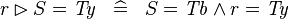$r \ranres S = \mathit{Ty} \;\;\defi\;\; S = \mathit{Tb} \land r = \mathit{Ty}$ where$\mathit{Ty}$ is a type expression equal to$\mathit{Ta} \cprod \mathit{Tb}$ A
*
SIMP_RANSUB_EQUAL_EMPTY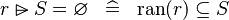$r \ransub S = \emptyset \;\;\defi\;\; \ran (r) \subseteq S$ A
*
SIMP_RANSUB_EQUAL_TYPE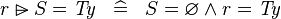$r \ransub S = \mathit{Ty} \;\;\defi\;\; S = \emptyset \land r = \mathit{Ty}$ where$\mathit{Ty}$ is a type expression A
*
SIMP_CONVERSE_EQUAL_EMPTY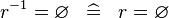$r^{-1} = \emptyset \;\;\defi\;\; r = \emptyset$ A
*
SIMP_CONVERSE_EQUAL_TYPE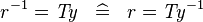$r^{-1} = \mathit{Ty} \;\;\defi\;\; r = \mathit{Ty}^{-1}$ where$\mathit{Ty}$ is a type expression A
*
SIMP_RELIMAGE_EQUAL_EMPTY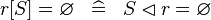$r[S] = \emptyset \;\;\defi\;\; S \domres r = \emptyset$ A
*
SIMP_OVERL_EQUAL_EMPTY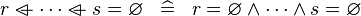$r \ovl \cdots \ovl s = \emptyset \;\;\defi\;\; r = \emptyset \land \cdots \land s = \emptyset$ A
*
SIMP_DPROD_EQUAL_EMPTY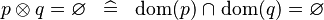$p \dprod q = \emptyset \;\;\defi\;\; \dom (p) \binter \dom (q) = \emptyset$ A
*
SIMP_DPROD_EQUAL_TYPE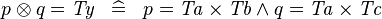$p \dprod q = \mathit{Ty} \;\;\defi\;\; p = \mathit{Ta} \cprod \mathit{Tb} \land q = \mathit{Ta} \cprod \mathit{Tc}$ where$\mathit{Ty}$ is a type expression equal to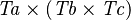$\mathit{Ta} \cprod (\mathit{Tb} \cprod \mathit{Tc})$ A
*
SIMP_PPROD_EQUAL_EMPTY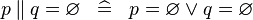$p \pprod q = \emptyset \;\;\defi\;\; p = \emptyset \lor q = \emptyset$ A
*
SIMP_PPROD_EQUAL_TYPE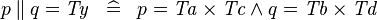$p \pprod q = \mathit{Ty} \;\;\defi\;\; p = \mathit{Ta} \cprod \mathit{Tc} \land q = \mathit{Tb} \cprod \mathit{Td}$ where$\mathit{Ty}$ is a type expression equal to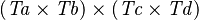$(\mathit{Ta} \cprod \mathit{Tb}) \cprod (\mathit{Tc} \cprod \mathit{Td})$ A
*
SIMP_ID_EQUAL_EMPTY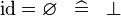$\id = \emptyset \;\;\defi\;\; \bfalse$ A
*
SIMP_PRJ1_EQUAL_EMPTY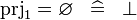$\prjone = \emptyset \;\;\defi\;\; \bfalse$ A
*
SIMP_PRJ2_EQUAL_EMPTY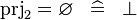$\prjtwo = \emptyset \;\;\defi\;\; \bfalse$ A